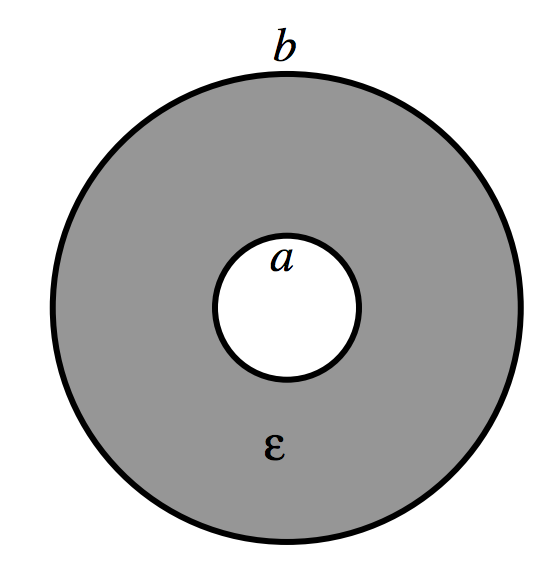$$\require{cancel}$$

# 5.3: Coaxial Cylindrical Capacitor

$$\newcommand{\vecs}{\overset { \rightharpoonup} {\mathbf{#1}} }$$ $$\newcommand{\vecd}{\overset{-\!-\!\rightharpoonup}{\vphantom{a}\smash {#1}}}$$$$\newcommand{\id}{\mathrm{id}}$$ $$\newcommand{\Span}{\mathrm{span}}$$ $$\newcommand{\kernel}{\mathrm{null}\,}$$ $$\newcommand{\range}{\mathrm{range}\,}$$ $$\newcommand{\RealPart}{\mathrm{Re}}$$ $$\newcommand{\ImaginaryPart}{\mathrm{Im}}$$ $$\newcommand{\Argument}{\mathrm{Arg}}$$ $$\newcommand{\norm}{\| #1 \|}$$ $$\newcommand{\inner}{\langle #1, #2 \rangle}$$ $$\newcommand{\Span}{\mathrm{span}}$$ $$\newcommand{\id}{\mathrm{id}}$$ $$\newcommand{\Span}{\mathrm{span}}$$ $$\newcommand{\kernel}{\mathrm{null}\,}$$ $$\newcommand{\range}{\mathrm{range}\,}$$ $$\newcommand{\RealPart}{\mathrm{Re}}$$ $$\newcommand{\ImaginaryPart}{\mathrm{Im}}$$ $$\newcommand{\Argument}{\mathrm{Arg}}$$ $$\newcommand{\norm}{\| #1 \|}$$ $$\newcommand{\inner}{\langle #1, #2 \rangle}$$ $$\newcommand{\Span}{\mathrm{span}}$$

The radii of the inner and outer cylinders are $$a$$ and $$b$$, and the permittivity between them is $$\epsilon$$.$$\text{FIGURE V.4}$$

Suppose that the two cylinders are connected to a battery so that the potential difference between them is $$V$$, and the charge per unit length on the inner cylinder is $$+\lambda \text{C m}^{-1}$$, and on the outer cylinder is $$-\lambda \text{C m}^{-1}$$. We have seen (Subsection 2.2.3) that the potential difference between the cylinders under such circumstances is $$\frac{\lambda}{2\pi \epsilon}\ln (b/a)$$. Therefore the capacitance per unit length, $$C'$$, is

$C'=\frac{2\pi \epsilon}{\ln (b/a)}.\label{5.3.1}$

This is by no means solely of academic interest. The capacitance per unit length of coaxial cable (“coax”) is an important property of the cable, and this is the formula used to calculate it.

This page titled 5.3: Coaxial Cylindrical Capacitor is shared under a CC BY-NC 4.0 license and was authored, remixed, and/or curated by Jeremy Tatum via source content that was edited to the style and standards of the LibreTexts platform; a detailed edit history is available upon request.